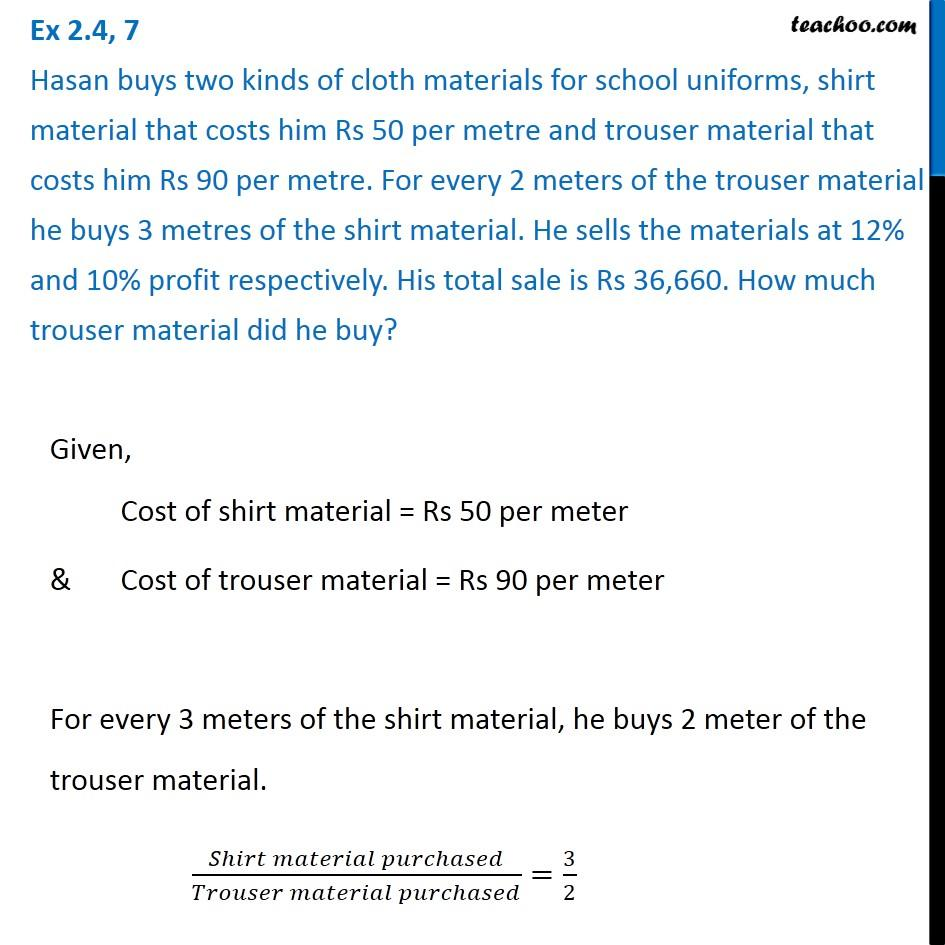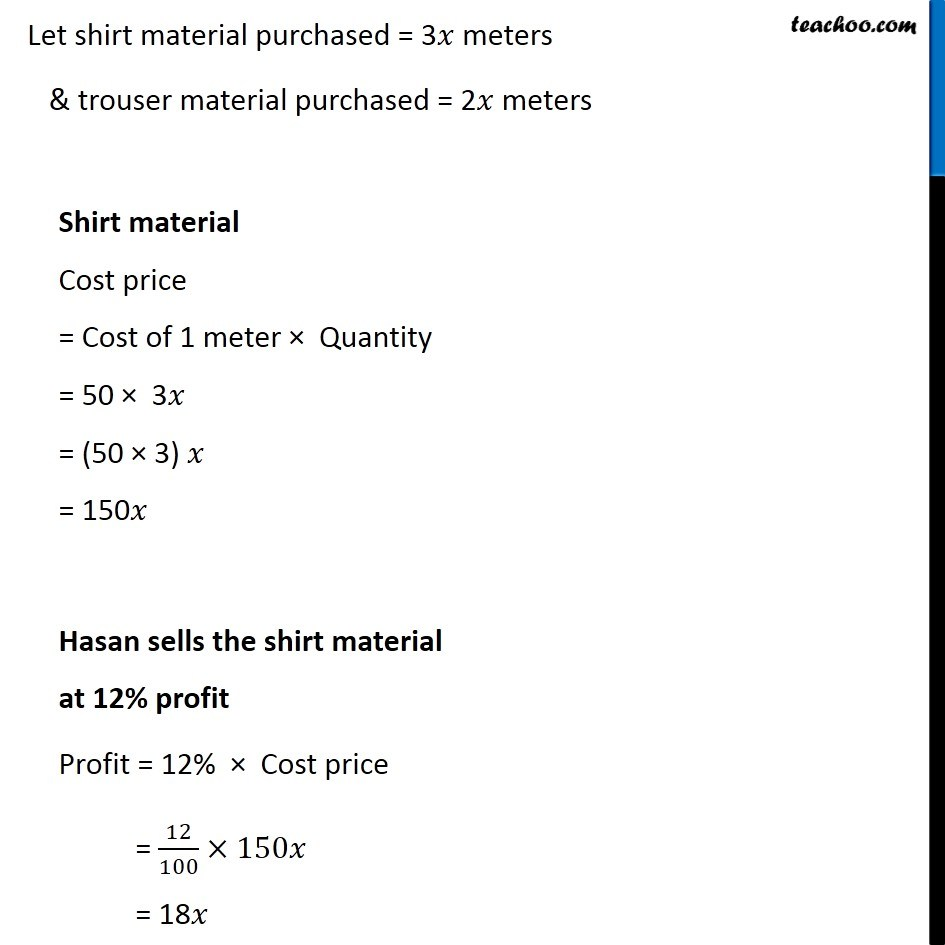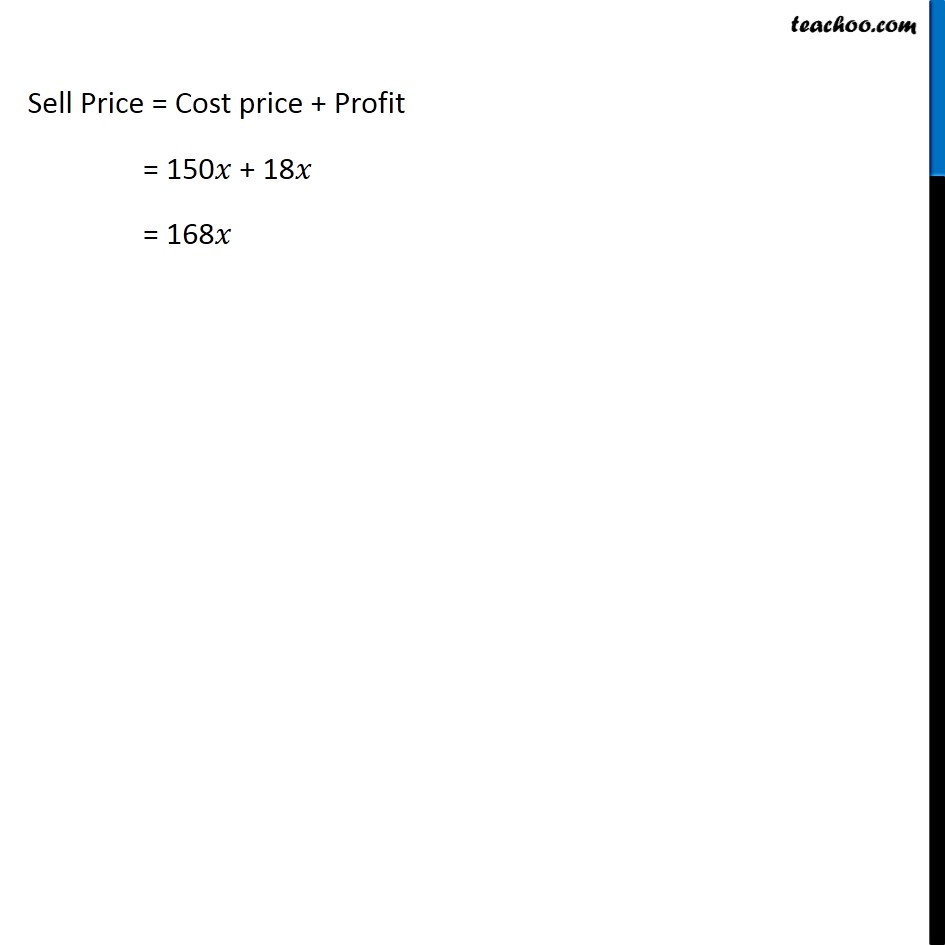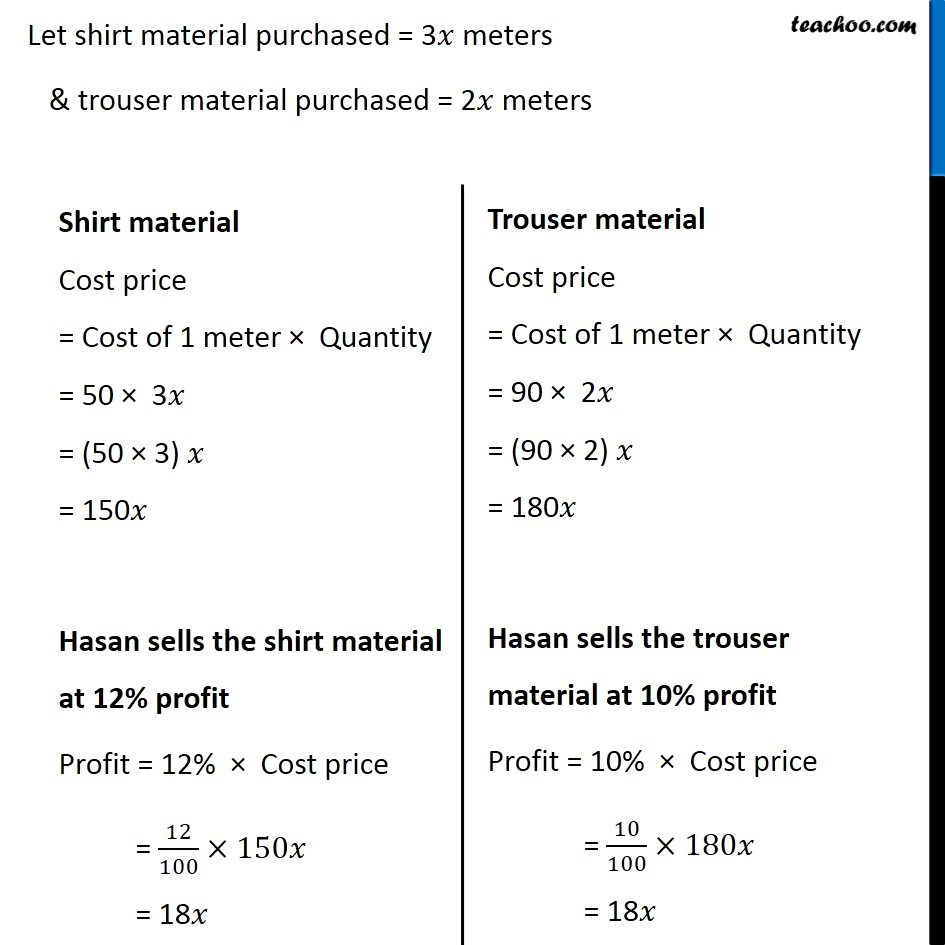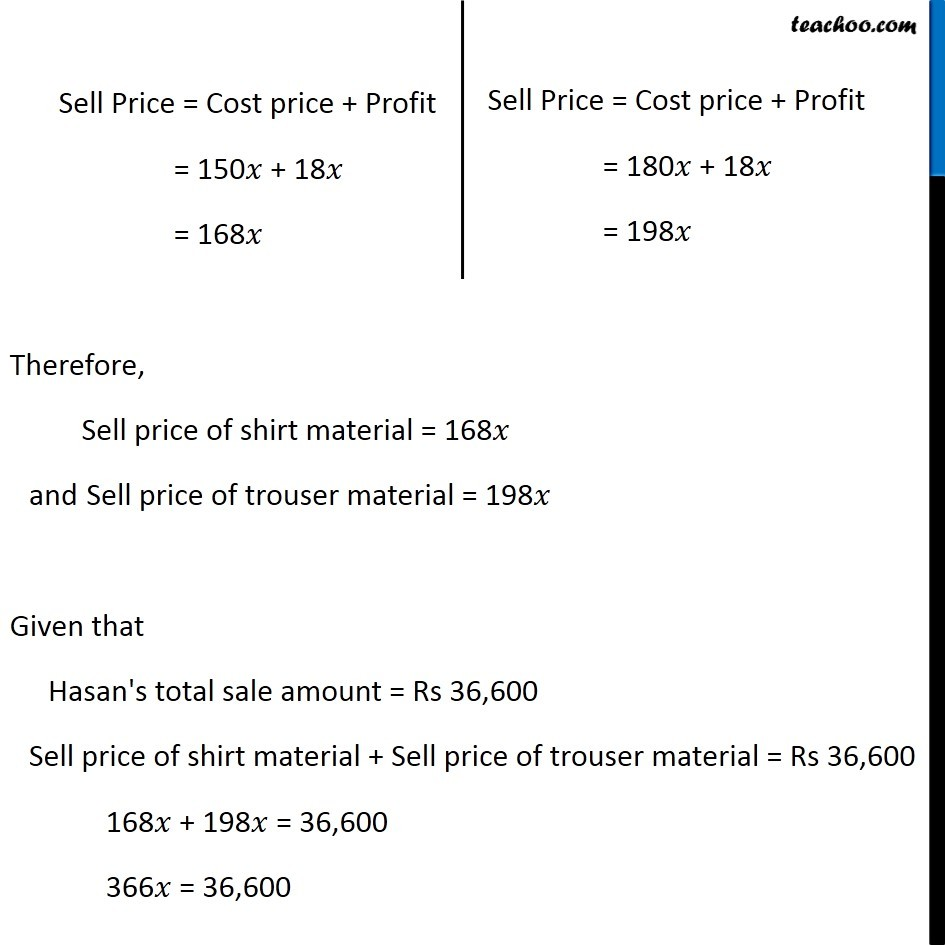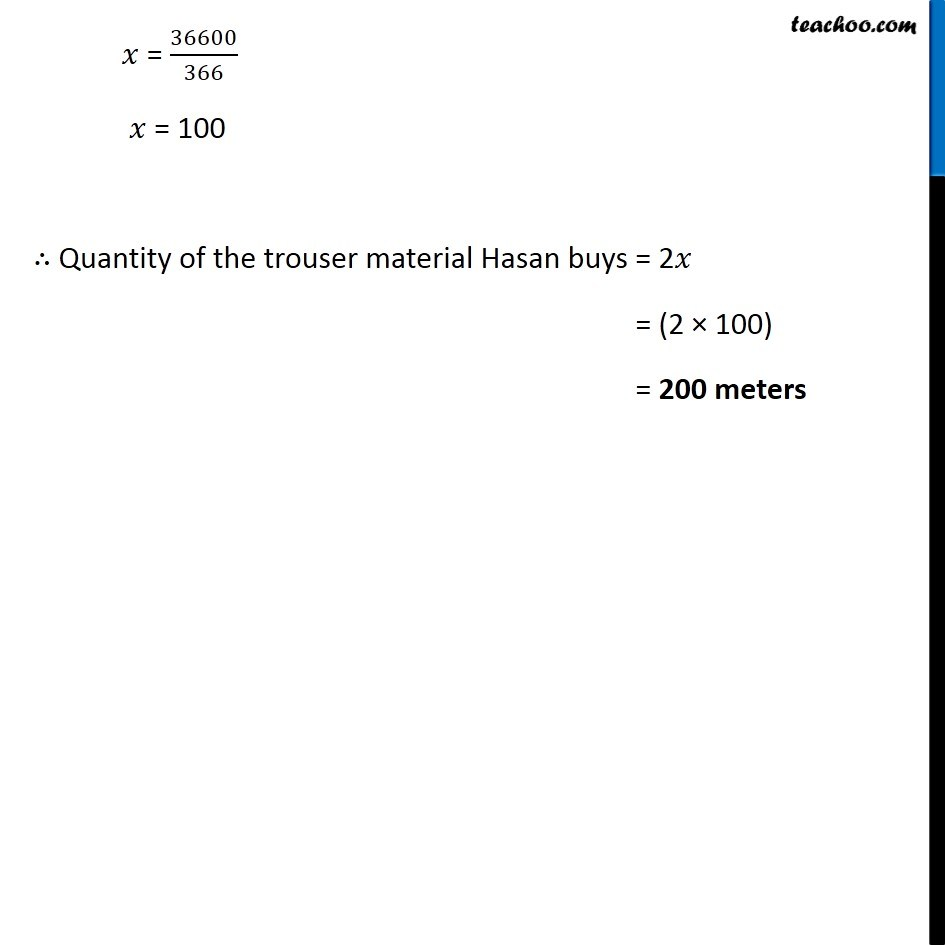1. Chapter 2 Class 8 Linear Equations in One Variable
2. Concept wise
3. Forming Linear Equations - Cost, Sale, Profit

Transcript

Ex 2.4, 7 Hasan buys two kinds of cloth materials for school uniforms, shirt material that costs him Rs 50 per metre and trouser material that costs him Rs 90 per metre. For every 2 meters of the trouser material he buys 3 metres of the shirt material. He sells the materials at 12% and 10% profit respectively. His total sale is Rs 36,660. How much trouser material did he buy?Given, Cost of shirt material = Rs 50 per meter & Cost of trouser material = Rs 90 per meter For every 3 meters of the shirt material, he buys 2 meter of the trouser material. (𝑆ℎ𝑖𝑟𝑡 𝑚𝑎𝑡𝑒𝑟𝑖𝑎𝑙 𝑝𝑢𝑟𝑐ℎ𝑎𝑠𝑒𝑑)/(𝑇𝑟𝑜𝑢𝑠𝑒𝑟 𝑚𝑎𝑡𝑒𝑟𝑖𝑎𝑙 𝑝𝑢𝑟𝑐ℎ𝑎𝑠𝑒𝑑)=3/2 Let shirt material purchased = 3𝑥 meters & trouser material purchased = 2𝑥 meters Shirt material Cost price = Cost of 1 meter × Quantity = 50 × 3𝑥 = (50 × 3) 𝑥 = 150𝑥 Hasan sells the shirt material at 12% profit Profit = 12% × Cost price = 12/100×150𝑥 = 18𝑥 Sell Price = Cost price + Profit = 150𝑥 + 18𝑥 = 168𝑥 Let shirt material purchased = 3𝑥 meters & trouser material purchased = 2𝑥 meters Shirt material Cost price = Cost of 1 meter × Quantity = 50 × 3𝑥 = (50 × 3) 𝑥 = 150𝑥 Hasan sells the shirt material at 12% profit Profit = 12% × Cost price = 12/100×150𝑥 = 18𝑥 Trouser material Cost price = Cost of 1 meter × Quantity = 90 × 2𝑥 = (90 × 2) 𝑥 = 180𝑥 Hasan sells the trouser material at 10% profit Profit = 10% × Cost price = 10/100×180𝑥 = 18𝑥 Trouser material Cost price = Cost of 1 meter × Quantity = 90 × 2𝑥 = (90 × 2) 𝑥 = 180𝑥 Hasan sells the trouser material at 10% profit Profit = 10% × Cost price = 10/100×180𝑥 = 18𝑥 Therefore, Sell price of shirt material = 168𝑥 and Sell price of trouser material = 198𝑥 Given that Hasan's total sale amount = Rs 36,600 Sell price of shirt material + Sell price of trouser material = Rs 36,600 168𝑥 + 198𝑥 = 36,600 366𝑥 = 36,600 𝑥 = 36600/366 𝑥 = 100 ∴ Quantity of the trouser material Hasan buys = 2𝑥 = (2 × 100) = 200 meters

Forming Linear Equations - Cost, Sale, Profit

About the AuthorDavneet Singh
Davneet Singh is a graduate from Indian Institute of Technology, Kanpur. He has been teaching from the past 9 years. He provides courses for Maths and Science at Teachoo.